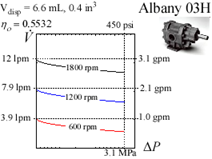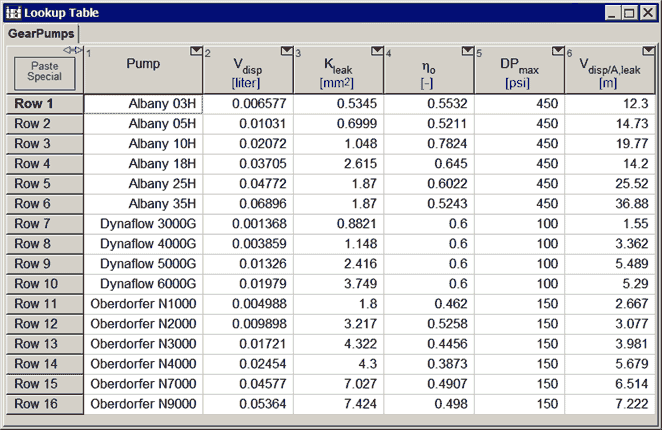Contents - index

Gearpump2_CLThe procedure Gearpump3 provides a model of a specific manufacturer's gear pump.  The parameters displacement, leakage constant, overall efficiency, and maximum DP are determined by curve fit to manufacturer's data and are stored in the Lookup Table 'GearPumps.lkt'.  An example of the data is shown in the thumbnail above, but there are many other sets of data to choose from.

Volumetric flow rate is determined from displacement less leakage flow.  Power is determined from overall efficiency applied to displacement flow.

Inputs:

F\$:  fluid string identifier

C:  concentration (%) {applicable when F\$ is a brine.  Otherwise set C=0.}

T_in:  inlet temperature (K, C, F, or R)

P_in:  inlet pressure (bar, atm, Pa, kPa, MPa)

P_out:  outlet pressure (bar, atm, Pa, kPa, MPa)

N:  rotational speed (1/s)

Pump\$:  pump model string identifier, which must be a string that appears in the first column of the GearPumpt.lkt table below. .Outputs:

m_dot:  mass flow rate (kg/s or lb_m/hr)

T_out:  outlet temperature (K, C, F, or R)

W_dot:  power (W, kW or Btu/hr)

eta_p:  actual efficiency relative to power

eta_v:  volumetric efficiency"

Example 1:

\$UnitSystem SI Mass kJ K Pa

F\$='DowTherm_J'

C=0 [%]

T_in=300 [K]

P_in=100000 [Pa]

P_out=500000 [Pa]

N=1700 [1/min]*convert(1/min,1/s)

Pump\$='Albany 05H'

Call gearpump2_cl(F\$, C, T_in, P_in, P_out, N, Pump\$: m_dot, T_out, W_dot, eta_p, eta_v)

{Solution:

eta_p = 0.4943

eta_v = 0.9485

m_dot = 0.240 [kg/s]

T_out = 25.25 [C]

W_dot = 0.2242 [kW]

}

Example 2:

\$UnitSystem SI Mass kJ C kPa

F\$='EG'

C=20 [%]

T_in=25 [C]

P_in=100 [kPa]

P_out=500 [kPa]

N=1700 [1/min]*convert(1/min,1/s)

Pump\$='Albany 05H'

Call gearpump2_cl(F\$, C, T_in, P_in, P_out, N, Pump\$: m_dot, T_out, W_dot, eta_p, eta_v)

{Solution:

eta_p = 0.4964

eta_v = 0.9526

m_dot = 0.2397 [kg/s]

T_out = 25.2 [C]

W_dot = 0.2242 [kW]

}